Office Applications and Entertaiment, Magic SquaresForm4b About the Author

Pan Magic Squares 4 x 4, Interactive Solution based on Binary Matrices

Introduction

Another well known construction method for Pan Magic Squares is based on the binary representation of numbers (0 = 0000,
1 = 0001, 2 = 0010, 3 = 0011 ... 15 = 1111).

Any Magic Square of the 4th order with the numbers 1 ... 16 can be written as B1 + 2 * B2 + 4 * B3 + 8 * B4 + , where the matrices B1, B2, B3 and B4 - further referred to as Binaries - contain only the numbers 0 and 1.

The 8 for Pan Magic Squares applicable Binaries are shown below in the bottom part of the form.

A 4th order Pan Magic Square can be constructed by means of the Matrix Operation PM = B1 + 2 * B2 + 4 * B3 + 8 * B4 + .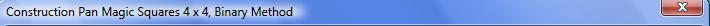Selected:

 B1B2B3B4Pan Magic: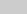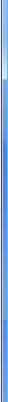Binaries: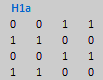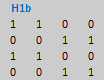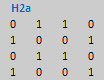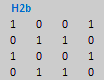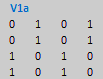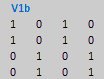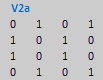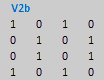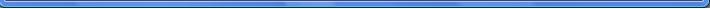Procedure:

1. Select the matrices B1, B2, B3 or B4 with the selection button left.

2. Select for each of the matrices one Binary from the pairs H1a/H1b, H2a/H2b, V1a/V1b and V2a/V2b by clicking the corresponding image shown in the bottom part of the form.

3. A selection can be cancelled by clicking subject image in the top part of the form, after which another Binary can be selected.

4. Press the button ‘Show Square’ to calculate and visualise the resulting Pan Magic Square.

All 384 Pan Magic Squares can be determined by means of this method as the 24 = 16 possible combinations and 4! = 24 permutations of the Binaries will result in 16 * 24 = 384 different Pan Magic Squares.

All combinations, permutations and resulting Pan Magic Squares of the 4th order are shown in Attachment 4b.

Have Fun!About the Author